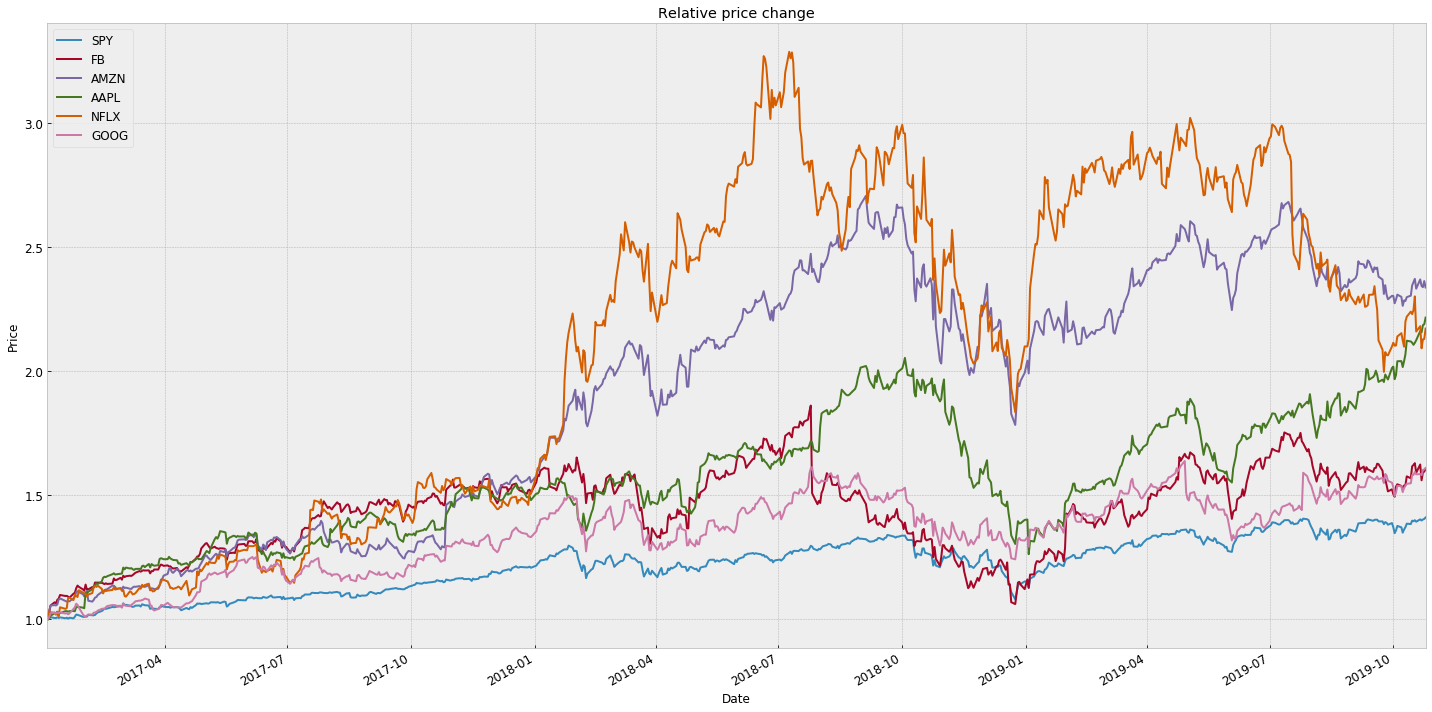Often times we need to compare stock performance between each other or against the index during specific time interval. Since different stocks have different price values, the comparison is done on relative basis, where all the prices are normalized to 1$at the first day of the time interval we are interested in. Very useful for comparing not only stocks between each other, but also major indexes (or ETFs) in the world. This way we can for example compare how is USA technology ETF (QQQ) doing against Chinese tech ETF (CHIK) for example. In this specific example we are comparing so called FAANG stocks (Facebook, Amazon, Apple, Netfix and Google ['FB','AMZN','AAPL','NFLX','GOOG']) to S&P500 ETF (SPY). In : #optional installations: #!pip install yfinance --upgrade --no-cache-dir #!pip3 install pandas_datareader #!pip3 install pandas --upgrade # better display from IPython.core.display import display, HTML display(HTML("<style>.container { width:100% !important; }</style>")) # ___library_import_statements___ import pandas as pd # for pandas_datareader, otherwise it might have issues, sometimes there is some version mismatch pd.core.common.is_list_like = pd.api.types.is_list_like import pandas_datareader.data as web import numpy as np import matplotlib.pyplot as plt import seaborn as sns import datetime import time #newest yahoo API import yfinance as yahoo_finance %matplotlib inline  Function definitions: In : def f_plot_selected(df, columns, start_index, end_index): """Plot the desired columns over index values in the given range.""" # takes a dataframe, columns (list) that we want to print and range of rows # note: df.ix is deprecated now f_plot_data(df.loc[start_index:end_index]) # use df.loc not df.iloc since we use datetime as index def f_get_data(symbols, dates, start_time, today): """Read stock data (adjusted close) for given symbols from yahoo finance""" df = pd.DataFrame(index=dates) print(df.head()) if 'SPY' not in symbols: # add SPY for reference, if absent symbols.insert(0, 'SPY') for symbol in symbols: # yahoo gives only daily historical data, more granular data stream is hard to get for free connected = False while not connected: try: ticker_df = web.get_data_yahoo(symbol, start=start_time, end=today) connected = True print('connected to yahoo') except Exception as e: print("type error, something is wrong: " + str(e)) time.sleep( 10 ) pass # reset index from dates to index numbers # print(ticker_df.head(2)) # Date High # 2017-01-03 128.190002 # 2017-01-04 130.169998 ticker_df = ticker_df.reset_index() # print(ticker_df.head(2)) # Date High # 0 2017-01-03 128.190002 # 1 2017-01-04 130.169998 ticker_df.set_index('Date', inplace=True, drop=False) df_temp = ticker_df[['Date','Adj Close']] df_temp = df_temp.rename(columns={'Adj Close': symbol}) df = df.join(df_temp[symbol]) if symbol == 'SPY': # drop dates SPY did not trade df = df.dropna(subset=["SPY"]) return df def f_normalize_data(df): """normalizes stock data in respect to price in day 1, this way price on the first day starts at 1$ for any given stock"""
#return df/df.ix[0,:]      # deprecated option
return df/df.iloc[0,:]     # use df.iloc not df.loc since index is number

def f_plot_data(df, title="Stock prices"):
"""Plot stock prices with a custom title and meaningful axis labels."""

ax = df.plot(title=title, fontsize=12, figsize=(20, 10))
ax.set_xlabel("Date")
ax.set_ylabel("Price")

#plt.figure(figsize=(20, 10), dpi= 120, facecolor='w', edgecolor='k')
plt.title('Relative price change')
plt.legend(loc='upper left', fontsize=12)
plt.tight_layout()
plt.style.use('bmh')
plt.grid(True)
plt.show()

def f_run():
# Define a date range
start_time = datetime.datetime(2017, 1, 1)
# end_time = datetime.datetime(2018, 6, 20)
today = datetime.datetime.now().date().isoformat()
dates = pd.date_range(start_time, today)

# Choose stock symbols to read
symbols = ['FB', 'AMZN', 'AAPL', 'NFLX', 'GOOG']
# SPY will be added in f_get_data() function automatically

# Get stock data
df = f_get_data(symbols, dates, start_time, today)
df = f_normalize_data(df)

# Slice and plot
f_plot_selected(df, ['SPY', 'FB', 'AMZN', 'AAPL', 'NFLX', 'GOOG'], start_time, today)


Final evaluation:

In :
if __name__ == "__main__":
f_run()

Empty DataFrame
Columns: []
Index: [2017-01-01 00:00:00, 2017-01-02 00:00:00, 2017-01-03 00:00:00, 2017-01-04 00:00:00, 2017-01-05 00:00:00]
connected to yahoo
connected to yahoo
connected to yahoo
connected to yahoo
connected to yahoo
connected to yahoo#### Source:¶

free Udacity course: Machine Learning for Trading, Lesson 3
https://eu.udacity.com/course/machine-learning-for-trading--ud501 code has been modified extensively